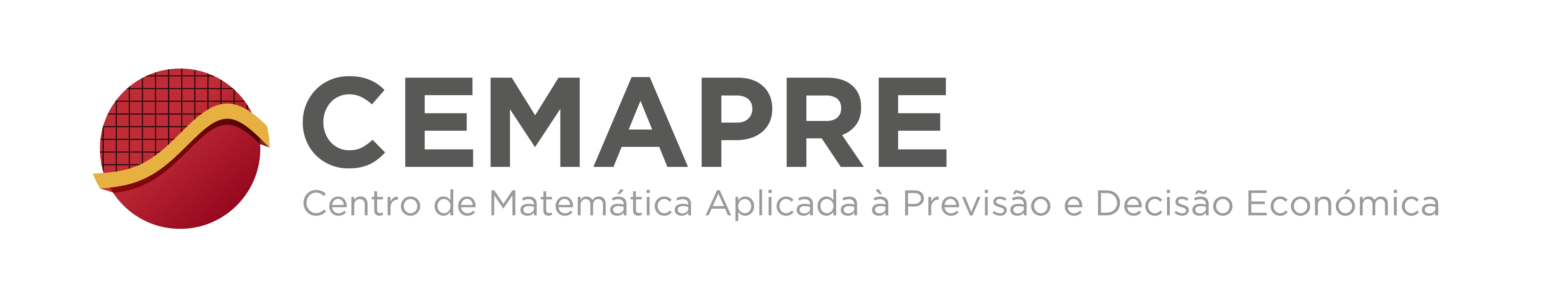## CEMAPRE Seminar

### Rotation number of contracted rotations

Arnaldo Nogueira
(Aix Marseille Université)

Abstract: Let $a\in(0,1)$, $0\leq b<1$ and $I=[0,1)$. We call contracted rotation the interval map $\varphi_{a,b} :x\in I \mapsto ax+b \pmod 1$. Once the parameter $a$ is fixed, we are interested in the family $\varphi_{a,b}$, where $b$ runs on the interval $I$. We use the fact that, as in the case of circle homeomorphisms, any contracted rotation $\varphi_{a,b}$ has a rotation number which depends only on the parameters $a$ and $b$. We will discuss the dynamical and diophantine aspects of the subject. In particular, we will show that, if $a$ and $b$ are algebraic numbers, the rotation number is rational using a transcendance theorem about the value of the Hecke-Mahler series at an algebraic point. The talk is based on a joint work with Michel Laurent.

Notice: Change of date
Wednesday, March 7, 2018
Time: 13h30
Room: Sala Delta, Edificio Quelhas, ISEG
http://cemapre.iseg.ulisboa.pt/seminars/cemapre/